# Division - 6th grade (11y) - math problems

#### Number of problems found: 252

• Milk cratesA farmer sells milk in crates that hold 15 bottles. She has 34,125 bottles of milk. How many crates can the farmer fill?
• Volume per timeHow long does fill take for a pump with a volume flow of 200 l per minute fill a cube-shaped tank up to 75% of its height if the length of the cube edge is 4 m?
• An electricianAn electrician needs 1 1/3 rolls of electrical wire to wire each room in a house. How many rooms can he wire with 6 2/3 rolls of wire?
• Illness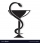Peter is ill and must take medication three times a day for one tablet. For how many days is a pack of 30 tablets enough for him, when on the third day he takes one tablet only in the morning and evening?
• PegsFrom two sticks 240 cm and 210 cm long, it is necessary to cut the longest possible pegs for flowers so that no residues remain. How many pegs will it be?
• The woodenThe wooden block measures 12 cm, 24 cm, and 30 cm. Peter wants to cut it into several identical cubes. At least how many cubes can he get?
• Into boxHow many cubes with an edge of 2.5 cm fit into a box measuring 11.6 cm; 8.9 cm and 13.75 cm?
• Zhiwei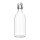Zhiwei wants to divide 19 litres of water equally into 6 pots. Find the volume of water in each pot?
• JagdeepJagdeep had a strip of paper measuring 1.8 m. He cut it into 4 equal strips. How long is each shorter strip of paper?
• Divisible by nineHow many three-digit natural numbers in total are divisible without a remainder by the number 9?
• Convalescent homes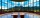In 270 convalescent homes, 94,270 vacationers spent part of the holiday a year. On average, how many vacationers per convalescent home?
• Thousand ballsWe have to create a thousand balls from a sphere with a diameter of 1 m. What will be their radius?
• Tennis balls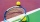Can of tennis balls contains 3 balls per can and cost \$7 how much will it cost for 36 tennis balls?
• RemainderWhat is the remainder of the division of natural numbers 293 and 7?
• Average priceSixth grade went on a trip to Moravia. Each of the 26 pupils paid CZK 320 and the school paid a total of CZK 3,510. What was the average price of a trip per student?
• Two sides paintThe door has the shape of a rectangle with dimensions of 260cm and 170cm. How many cans of paint will be needed to paint this door if one can of paint cover 2m2 of the area? We paint the doors on both sides.
• My fatherMy father cut 78 slats on the fence. The shortest of them was 97 cm long, the longer one was 102 cm long. What was the total length of the slats in cm?
• Identical cubesFrom the smallest number of identical cubes whose edge length is expressed by a natural number, can we build a block with dimensions 12dm x 16dm x 20dm?
• Largest squaresHow many of the largest square sheets did the plumber cut the honeycomb from 16 dm and 96 dm?
• The excavation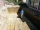The excavation for the city sewer was 38 m long, 2.2 m wide, and 3 m deep. a) How many cubic meters of soil were dredged b) How many journeys had to be made by one car when removing the soil, if they loaded an average of 4.5 m of cubic soil on each journe

Do you have an interesting mathematical word problem that you can't solve it? Submit a math problem, and we can try to solve it.

We will send a solution to your e-mail address. Solved examples are also published here. Please enter the e-mail correctly and check whether you don't have a full mailbox.

Please do not submit problems from current active competitions such as Mathematical Olympiad, correspondence seminars etc...